# M*N Puzzle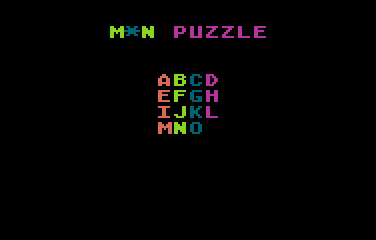This is a little game for the 2016 NOMAM's BASIC 10-liners Contest. This program fits in the PUR-80 category, and it was written using TurboBASIC XL 1.5 for the 8-bits ATARI XL/XE. Development started on 2016-02-25, and it took 4 days. The final version's date is 2016-03-03.

UPDATE: It obtained the 7th place of 46 entries in the category.

## Description

This is the classical 4x4 sliding puzzle with an empty space. To solve, just slide one piece at a time, trying to sort them alphabetically in columns and rows. Try to solve it with the minimum number of movements.

## Instructions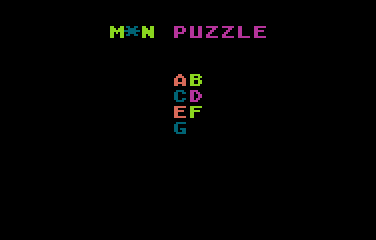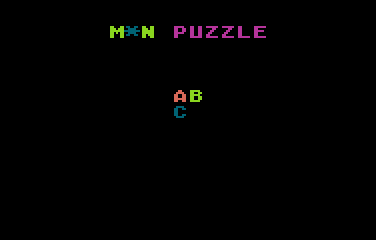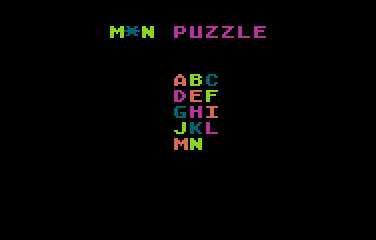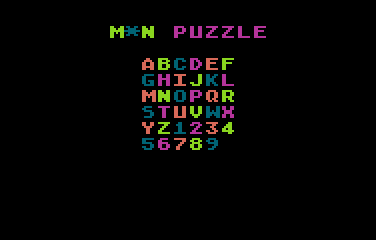Select the size of the puzzle using the joystick. Left and right decreases and increased the width, up and down decreases and increases the height.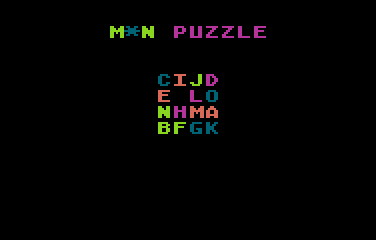Press the button to shuffle the selected puzzle.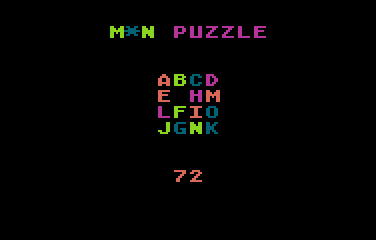Use the joystick to move a piece to the empty space. Up moves the piece that is bellow the space, left moves the piece at the right of it. The count of movements appears bellow the puzzle.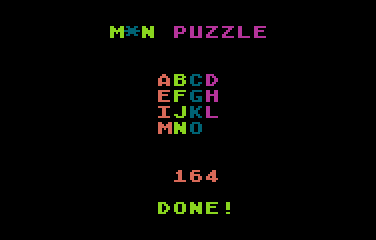When completed, you will hear some whistles... Press the button to select a new puzzle and play again.

## Development of the game

I was thinking for some days (in shower time) about a game that should fit the PUR-80 category. Finally, I sat down on Feb 26th in front of an editor and started. This game took me about half an hour of programming to confirm it should fit in 800 bytes of abbreviated BASIC code... actually it took less than 400!!! BTW, I used my own tool to parse the BASIC code and create a abbreviated listing for the Atari (*).

Then I spent more than 3 hours through the following days adding sound and special effects and the routine to select the size of the puzzle (initially, the size was required using INPUT), and arranging the code to follow the rules of the contest.

Finally, while writing this page which took me many hours, I found and remove a couple of bugs I introduced trying to make it more playable... Enjoy it!

(*) My little perl tool is very limited in functionalities, but I like it because it does what I need in the way I want. If you'd like to try one of these tools, I'd suggest DMSC's TurboBasic XL Parser Tool.

Get the MNPUZZLE.ATR file and set it as drive 1 in a real Atari or emulator. Turn on the computer and the game should start after loading. A joystick in port 1 is required.

## The code

The abbreviated BASIC code is the following: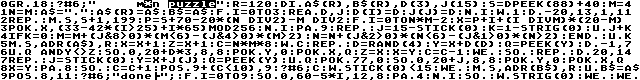The full and expanded BASIC listing is:

 ```GRAPHICS 18 ? #6;" M*N PUZZLE" ``` Initializes the screen and prints the game title. Uses standard graphics mode 2 (big text) without normal text window at the bottom, and default colours. ```R=120 DIM A\$(R),B\$(R),D(3),J(15) S=DPEEK(88)+40 M=4 N=M A\$="." A\$(R)=A\$ B\$=A\$ ``` Initializes constants and variables:R is the the length of the screen area where the maze will be placed.S is the memory location of the playfield.M is the width and N is the height, starting at 4x4.A\$ has a copy of the playfield containing the solved puzzle, and B\$ has a copy of the current playfield during the game. Both string variables need to be of the same maximum size R for the string comparison to work.D() and J() arrays store movement information as follows... ```FOR I=0 TO 3 READ D,J D(I)=D J(J)=D NEXT I DATA -20,13,1,11 DATA -1,7 DATA 20,14 ``` Set up screen deltas for random and joystick movements for all four directions. Graphics mode 2 uses 20 bytes per line, then you have to substract 20 bytes to find the screen position of the piece in the upper side of the current screen position, and add 1 to find the one at the right.D(0-3) array stores the four deltas for random shufleJ(0-15) store the same four deltas for UP, RIGHT, LEFT and DOWN joystick positions and zero ("dont' move") for the other positions (default values in TurboBASIC XL).Data instructions were placed at the end of other lines to save space. ```WHILE 1 ``` End-less loop... ``` REPEAT ``` Begin of the routine to select the size of the puzzle, from 2x2 up to 6x6. ``` MOVE S, S+1, 199 ``` Clears playfield area. ``` P = S + 70 - 20*(N DIV 2) - M DIV 2 ``` Finds the upper left position in screen area of the puzzle for the current size of it. ``` FOR I=0 TO N*M-2 X=P+I+(I DIV M)*(20-M) POKE X, (33-42*(I>25)+I*65) MOD 256 NEXT I ``` Draws the puzzle, except the last piece.X is the screen position of the current piece.The pieces starts alphabetically from A to Z. In sizes that require more than 26 pieces, numbers 1 to 9 are included, giving 35 pieces for the largest 6x6 mode (including one empty space).At the same time, it asigns a colour for every piece, obtaining a different pattern based on current puzzle size. ``` PAUSE 9 ``` Just a pause before accepting a change of the size. If there is no pause, it's possible to change the size two times in just one move. ``` REPEAT J=15-STICK(0) K=1-STRIG(0) UNTIL J+K ``` Waits until joystick is moved or button is pressed. Bits are being negated to be more useful: 0=released, 1=pressed for button and one bit per direction for joystick. ``` IF K=0 M=M+(J&8>0)*(M<6)-(J&4>0)*(M>2) N=N+(J&2>0)*(N<6)-(J&1>0)*(N>2) ENDIF ``` Based on the joystick movement, changes the width and/or height of the puzzle. Diagonal movement is allowed!M increases by one only if the right bit of the joystick is set and the current size is less than the maximum allowed size, and decreased only if the left bit is set and current size is more than the minimum allowed size. The same for N in the other axis... BTW, all this happens only if the button was not pressed. ``` UNTIL K ``` Quits the game setup routine when the button is pressed. ``` MOVE S,ADR(A\$),R ``` Saves the screen data for the solved puzzle in A\$. ``` X=X+1 Z=X+1 C=N*M*8 ``` Sets the current position as the empty cell in X, the previous position in Z (fake for the first time), and the number of moves required to shuffle the puzzle in C, based on the selected size of the puzzle. ``` WHILE C ``` Begin of the shuffling routine. ``` REPEAT D=RAND(4) Y=X+D(D) Q=PEEK(Y) UNTIL Q AND Y<>Z ``` Randomly select a direction to find a piece to be moved to the empty space. Q is the piece, Y is it's position on screen. It shouldn't be the same piece from the previous move. ``` SOUND 0,20+D*3,8,8 ``` Plays a sound. The tone is based on the selected direction. ``` POKE Y,0 POKE X,Q ``` Move the selected piece to the empty position. ``` Z=X X=Y C=C-1 ``` Sets the position of the last moved piece, the new new position of the empty space and decreases the pending move's counter. ``` WEND SOUND ``` End of the shufling routine. Needs to turn off shuffling sound. ``` REPEAT ``` Begin of the gameplay routine. ``` REPEAT J=STICK(0) Y=X+J(J) Q=PEEK(Y) UNTIL Q ``` Select the piece to move based on the joystick position. Note that joystick movement is inverted: UP moves the empty space down, i.e. moves up the piece under it. There are no pieces neither in the empty position (joystick in released position) nor outside the puzzle area. ``` POKE 77,0 ``` Clears the attract mode register. This prevents the change of screen colours while playing, or restore the screen colours after a long pause while playing. ``` SOUND 0,20+J,8,8 ``` Plays a sound, the tone is based on the joystick position. ``` POKE Y,0 POKE X,Q X=Y ``` Move the selected piece to the empty space and set in X the new position of the empty space. ``` PAUSE 8 SOUND ``` Stop the sound after a small pause. ``` C=C+1 POSITION 9+(C<10),9 ? #6;C ``` Increase and print the count of movements. ``` WHILE STICK(0)<15 WEND ``` Waits until joystick is released. No continuous moves in this game. ``` MOVE S,ADR(B\$),R ``` Copies the current playfield to B\$. ``` UNTIL B\$=A\$ ``` Compares the current payfield against the solved one. If they match, the game ends. ``` POSITION 8,11 ? #6; "DONE!"; FOR I=0 TO 9 SOUND 0,60-5*I,12,8 PAUSE 4 NEXT I SOUND ``` Congratulations! You solved the puzzle. Bells and whistles... ``` WHILE STRIG(0) WEND ``` Wait for the button to be pressed to start again. ```WEND ``` Restart, preserving the current puzzle size, but it's possible to change it.